# 特征工程系列：时间特征构造以及时间序列特征构造

## 0x00 前言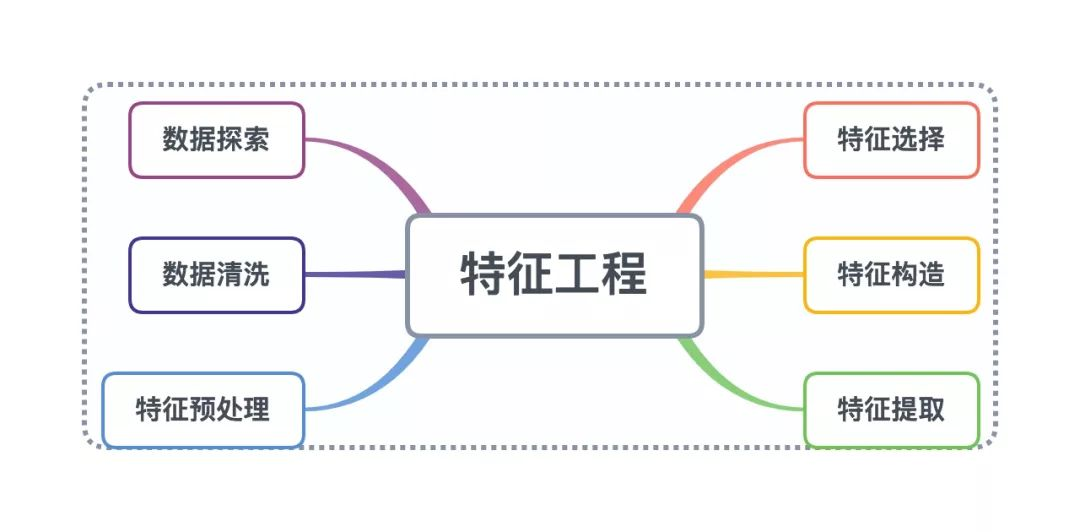——Andrew Ng

## 0x01 特征构造介绍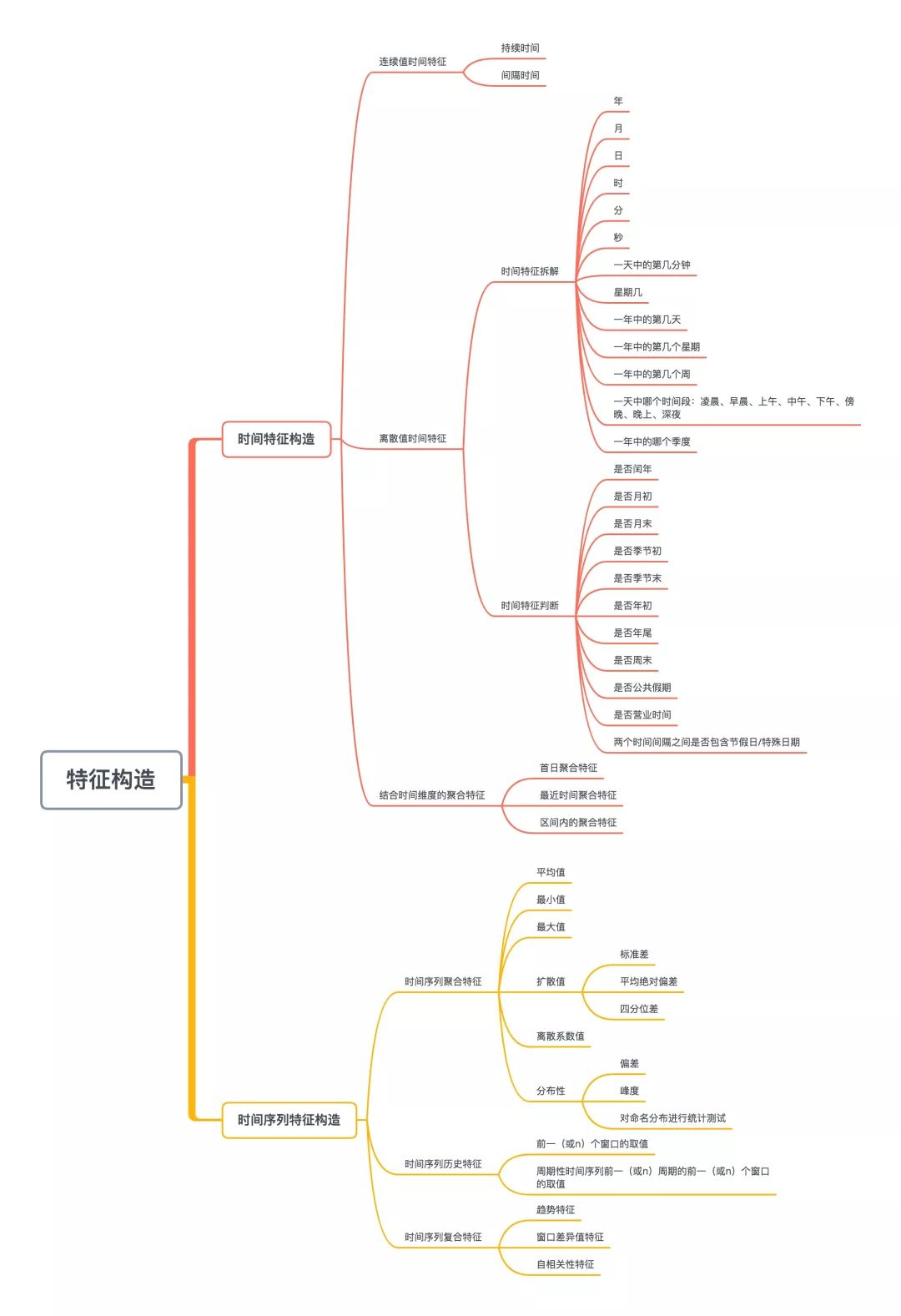## 0x02 时间特征构造

### 1.连续值时间特征

• 持续时间（单页浏览时长）；

• 间隔时间；

• 上次购买/点击离现在的时长；

• 产品上线到现在经过的时长；

### 2.离散值时间特征

#### 1）时间特征拆解

• 年；

• 月；

• 日；

• 时；

• 分；

• 数；

• 一天中的第几分钟；

• 星期几；

• 一年中的第几天；

• 一年中的第几个周；

• 一天中哪个时间段：凌晨、早晨、上午、中午、下午、傍晚、晚上、深夜；

• 一年中的哪个季度；

##### 程序实现
import pandas as pd# 构造时间数据date_time_str_list = [    '2019-01-01 01:22:26', '2019-02-02 04:34:52', '2019-03-03 06:16:40',    '2019-04-04 08:11:38', '2019-05-05 10:52:39', '2019-06-06 12:06:25',    '2019-07-07 14:05:25', '2019-08-08 16:51:33', '2019-09-09 18:28:28',    '2019-10-10 20:55:12', '2019-11-11 22:55:12', '2019-12-12 00:55:12',]df = pd.DataFrame({'时间': date_time_str_list})# 把字符串格式的时间转换成Timestamp格式df['时间'] = df['时间'].apply(lambda x: pd.Timestamp(x))# 年份df['年']=df['时间'].apply(lambda x: x.year)# 月份df['月']=df['时间'].apply(lambda x: x.month)# 日df['日']=df['时间'].apply(lambda x: x.day)# 小时df['时']=df['时间'].apply(lambda x: x.hour)# 分钟df['分']=df['时间'].apply(lambda x: x.minute)# 秒数df['秒']=df['时间'].apply(lambda x: x.second)# 一天中的第几分钟df['一天中的第几分钟']=df['时间'].apply(lambda x: x.minute + x.hour*60)# 星期几；df['星期几']=df['时间'].apply(lambda x: x.dayofweek)# 一年中的第几天df['一年中的第几天']=df['时间'].apply(lambda x: x.dayofyear)# 一年中的第几周df['一年中的第几周']=df['时间'].apply(lambda x: x.week)# 一天中哪个时间段：凌晨、早晨、上午、中午、下午、傍晚、晚上、深夜；period_dict ={    23: '深夜', 0: '深夜', 1: '深夜',    2: '凌晨', 3: '凌晨', 4: '凌晨',    5: '早晨', 6: '早晨', 7: '早晨',    8: '上午', 9: '上午', 10: '上午', 11: '上午',    12: '中午', 13: '中午',    14: '下午', 15: '下午', 16: '下午', 17: '下午',    18: '傍晚',    19: '晚上', 20: '晚上', 21: '晚上', 22: '晚上',}df['时间段']=df['时'].map(period_dict)# 一年中的哪个季度season_dict = {    1: '春季', 2: '春季', 3: '春季',    4: '夏季', 5: '夏季', 6: '夏季',    7: '秋季', 8: '秋季', 9: '秋季',    10: '冬季', 11: '冬季', 12: '冬季',}df['季节']=df['月'].map(season_dict)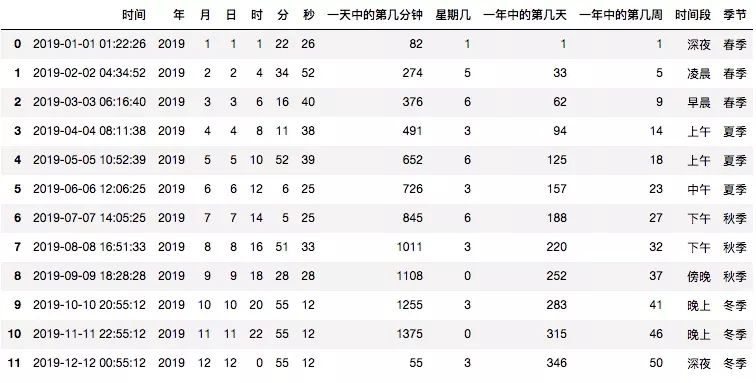#### 2）时间特征判断

• 是否闰年；

• 是否月初；

• 是否月末；

• 是否季节初；

• 是否季节末；

• 是否年初；

• 是否年尾；

• 是否周末；

• 是否公共假期；

• 是否营业时间；

• 两个时间间隔之间是否包含节假日/特殊日期；

##### 程序实现
import pandas as pd# 构造时间数据date_time_str_list = [    '2010-01-01 01:22:26', '2011-02-03 04:34:52', '2012-03-05 06:16:40',    '2013-04-07 08:11:38', '2014-05-09 10:52:39', '2015-06-11 12:06:25',    '2016-07-13 14:05:25', '2017-08-15 16:51:33', '2018-09-17 18:28:28',    '2019-10-07 20:55:12', '2020-11-23 22:55:12', '2021-12-25 00:55:12',    '2022-12-27 02:55:12', '2023-12-29 03:55:12', '2024-12-31 05:55:12',]df = pd.DataFrame({'时间': date_time_str_list})# 把字符串格式的时间转换成Timestamp格式df['时间'] = df['时间'].apply(lambda x: pd.Timestamp(x))# 是否闰年df['是否闰年'] = df['时间'].apply(lambda x: x.is_leap_year)# 是否月初df['是否月初'] = df['时间'].apply(lambda x: x.is_month_start)# 是否月末df['是否月末'] = df['时间'].apply(lambda x: x.is_month_end)# 是否季节初df['是否季节初'] = df['时间'].apply(lambda x: x.is_quarter_start)# 是否季节末df['是否季节末'] = df['时间'].apply(lambda x: x.is_quarter_end)# 是否年初df['是否年初'] = df['时间'].apply(lambda x: x.is_year_start)# 是否年尾df['是否年尾'] = df['时间'].apply(lambda x: x.is_year_end)# 是否周末df['是否周末'] = df['时间'].apply(lambda x: True if x.dayofweek in [5, 6] else False)# 是否公共假期public_vacation_list = [    '20190101', '20190102', '20190204', '20190205', '20190206',    '20190207', '20190208', '20190209', '20190210', '20190405',    '20190406', '20190407', '20190501', '20190502', '20190503',    '20190504', '20190607', '20190608', '20190609', '20190913',    '20190914', '20190915', '20191001', '20191002', '20191003',    '20191004', '20191005', '20191006', '20191007',] # 此处未罗列所有公共假期df['日期'] = df['时间'].apply(lambda x: x.strftime('%Y%m%d'))df['是否公共假期'] = df['日期'].apply(lambda x: True if x in public_vacation_list else False)# 是否营业时间df['是否营业时间'] = Falsedf['小时']=df['时间'].apply(lambda x: x.hour)df.loc[((df['小时'] >= 8) & (df['小时'] < 22)), '是否营业时间'] = Truedf.drop(['日期', '小时'], axis=1, inplace=True)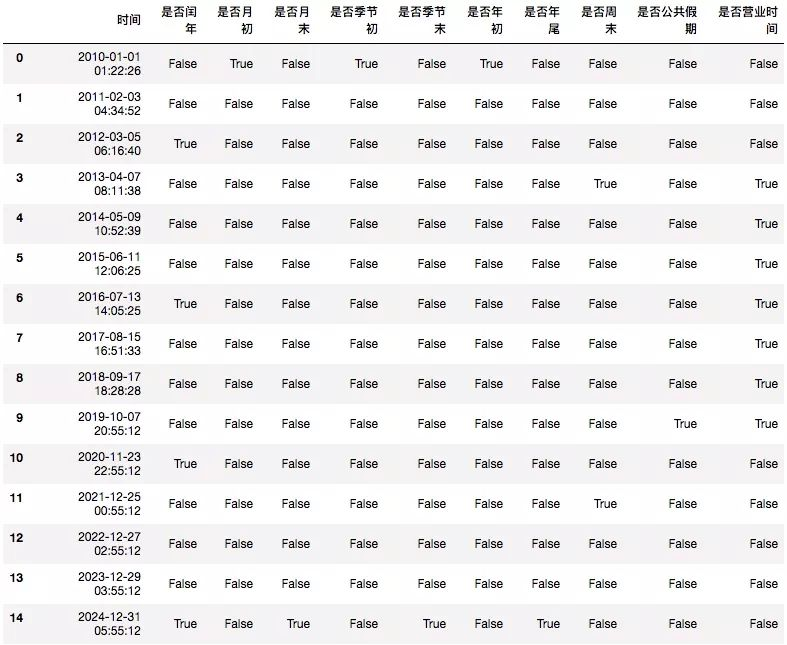## 0x03 时间序列特征构造

### 1.时间序列聚合特征

##### 6）分布性

import pandas as pd# 加载洗发水销售数据集df = pd.read_csv('shampoo-sales.csv')df.dropna(inplace=True)df.rename(columns={'Sales of shampoo over a three year period': 'value'}, inplace=True)# 平均值mean_v = df['value'].mean()print('mean: {}'.format(mean_v))# 最小值min_v = df['value'].min()print('min: {}'.format(min_v))# 最大值max_v = df['value'].max()print('max: {}'.format(max_v))# 扩散值：标准差std_v = df['value'].std()print('std: {}'.format(std_v))# 扩散值：平均绝对偏差mad_v = df['value'].mad()print('mad: {}'.format(mad_v))# 扩散值：四分位差q1 = df['value'].quantile(q=0.25)q3 = df['value'].quantile(q=0.75)irq = q3 - q1print('q1={}, q3={}, irq={}'.format(q1, q3, irq))# 离散系数variation_v = std_v/mean_vprint('variation: {}'.format(variation_v))# 分布性：偏态系数skew_v = df['value'].skew()print('skew: {}'.format(skew_v))# 分布性：峰态系数kurt_v = df['value'].kurt()print('kurt: {}'.format(kurt_v))# 输出：mean: 312.59999999999997min: 119.3max: 682.0std: 148.93716412347473mad: 119.66666666666667q1=192.45000000000002, q3=411.1, irq=218.65variation: 0.47644646232717447skew: 0.8945388528534595kurt: 0.11622821118738624

• 上面是单个时间序列的实现代码，多个时间序列的数据集构造特征时需要先进行分组再计算。如IJCAI-17口碑商家客流量预测比赛中，数据集中包含多个商家的历史销售数据，构造特征时需要先按商家分组，然后再构建特征。

• 上述代码都是使用所有历史数据来构造特征，实际项目中如果待预测目标为t时刻的值，则使用t时刻之前的值来构造特征，不同的t值都可以分别构造训练样本对应的特征。
如：使用t时刻的y值作为label，则使用t-1时刻之前的y值来构造特征；使用t-1时刻的y值作为label时，则使用t-2时刻之前的y值来构造特征。如此类推，我们可以得到多个训练样本，每个样本有多个特征。

### 2.时间序列历史特征

#### 2）周期性时间序列前一（或n）周期的前一（或n）个窗口的取值

import pandas as pd# 加载洗发水销售数据集df = pd.read_csv('shampoo-sales.csv')df.dropna(inplace=True)df.rename(columns={'Sales of shampoo over a three year period': 'value'}, inplace=True)df['-1day'] = df['value'].shift(1)df['-2day'] = df['value'].shift(2)df['-3day'] = df['value'].shift(3)df['-1period'] = df['value'].shift(1*12)df['-2period'] = df['value'].shift(2*12)display(df.head(60))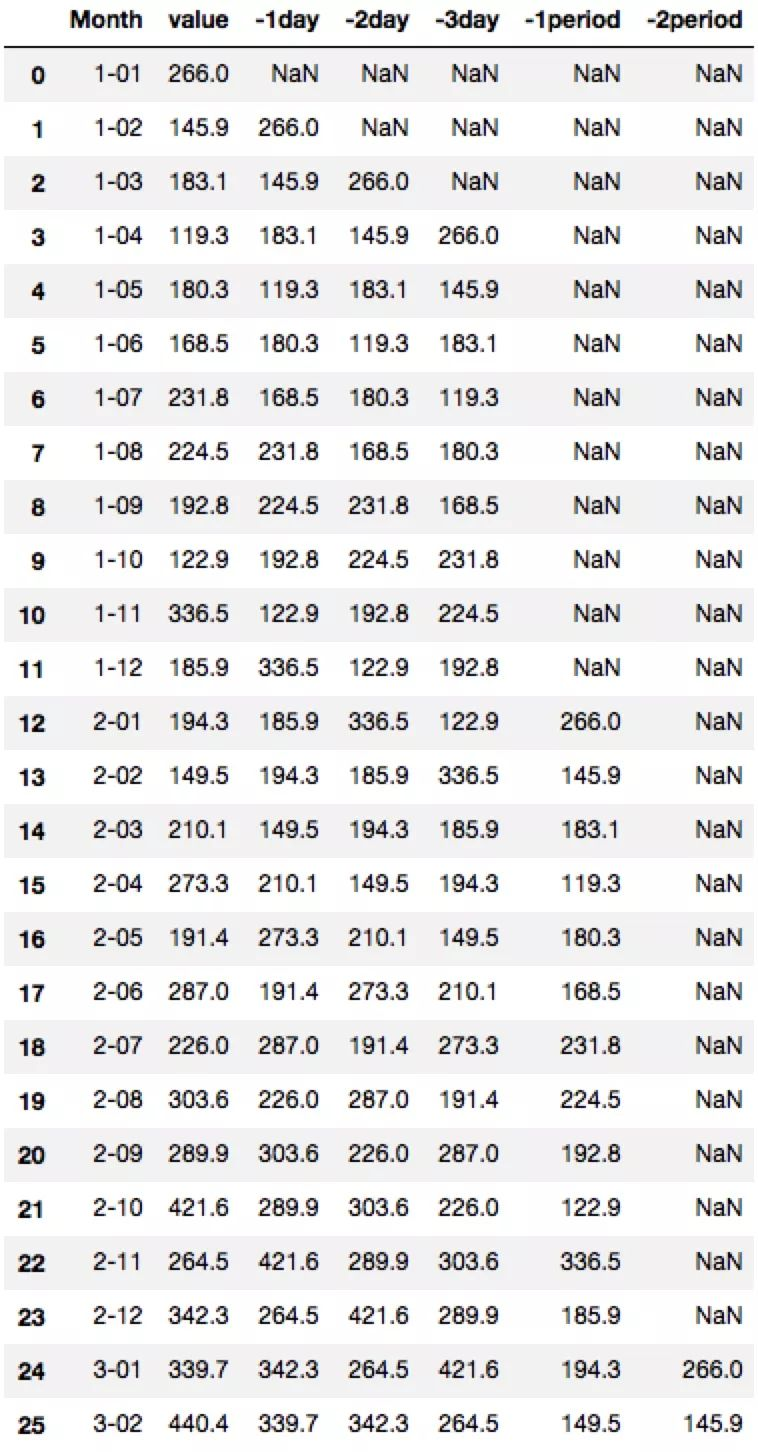### 3.时间序列复合特征

#### 1）趋势特征

import pandas as pd# 加载洗发水销售数据集df = pd.read_csv('shampoo-sales.csv')df.dropna(inplace=True)df.rename(columns={'Sales of shampoo over a three year period': 'value'}, inplace=True)df['last 3 day mean'] = (df['value'].shift(1) + df['value'].shift(2) + df['value'].shift(3))/3df['最近3天趋势'] = df['value'].shift(1)/df['last 3 day mean']display(df.head(60))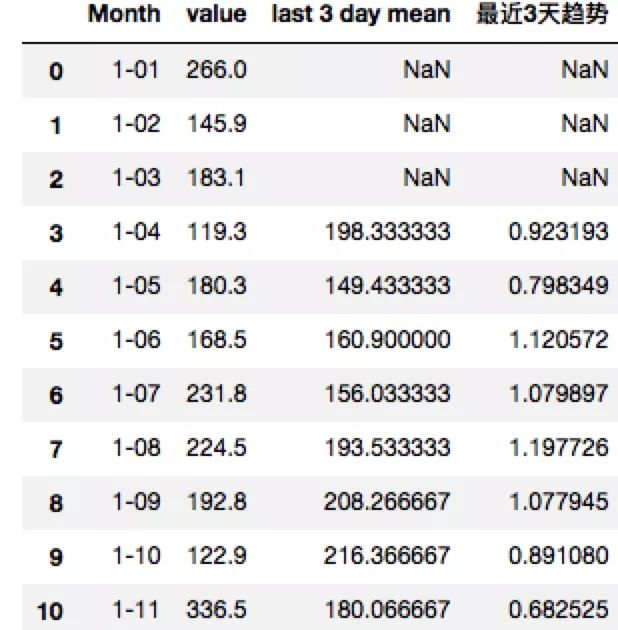#### 2）窗口差异值特征

import pandas as pd# 加载洗发水销售数据集df = pd.read_csv('shampoo-sales.csv')df.dropna(inplace=True)df.rename(columns={'Sales of shampoo over a three year period': 'value'}, inplace=True)df['最近两月销量差异值'] = df['value'].shift(1) - df['value'].shift(2)display(df.head(60))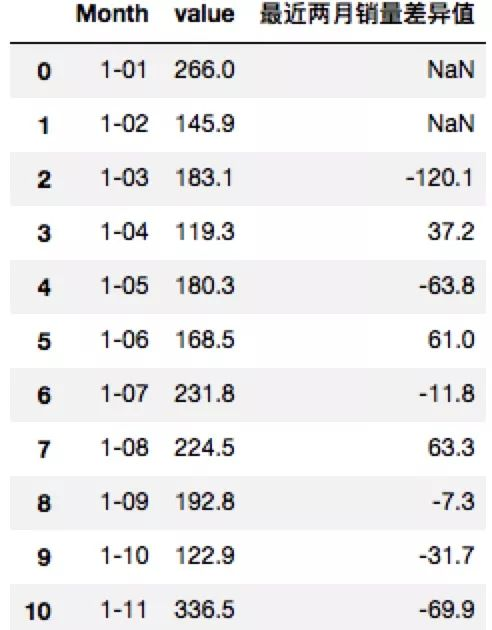#### 3）自相关性特征

import statsmodels.tsa.api as smtimport pandas as pd# 加载洗发水销售数据集df = pd.read_csv('shampoo-sales.csv')df.dropna(inplace=True)df.rename(columns={'Sales of shampoo over a three year period': 'value'}, inplace=True)print('滞后数为1的自相关系数：{}'.format(df['value'].autocorr(1)))print('滞后数为2的自相关系数：{}'.format(df['value'].autocorr(2)))# 输出：滞后数为1的自相关系数：0.7194822398024308滞后数为2的自相关系数：0.8507433352850972

## 0x0FF 总结

### 1.时间特征主要有两大类：

#### 1）从时间变量提取出来的特征

• 如果每条数据为一条训练样本，时间变量提取出来的特征可以直接作为训练样本的特征使用。

• 如果每条数据不是一条训练样本，时间变量提取出来的特征需要进行二次加工（聚合操作）才能作为训练样本的特征使用。

#### 参考文献

 https://www.cnblogs.com/nxf-rabbit75/p/11141944.html#_nav_12
 利用 gplearn 进行特征工程. https://bigquant.com/community/t/topic/120709
 Feature Tools：可自动构造机器学习特征的Python库. https://www.jiqizhixin.com/articles/2018-06-21-2
 各种聚类算法的系统介绍和比较. https://blog.csdn.net/abc200941410128/article/details/78541273

AI研发工程师成长指南08-30511712-032万+
05-201万+
03-177079
12-234262
09-071万+
10-131333
12-231万+
09-065269
01-261万+